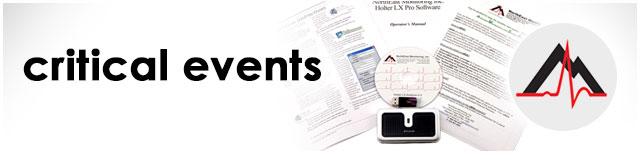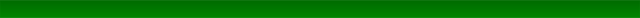For Heart Event Monitoring, How Fast Is “Fast Enough?”

There is a standing joke around the design-review table. During a heated design review, the wise, experienced engineer draws the Euler diagram below and says, “Pick two.”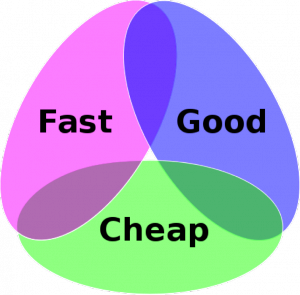Pick two.

The discussion today is about Fast. How fast is fast enough when it comes to sample rates for ECG recordings? We have an analog signal (ECG) that is being sampled and digitized; how fast do we need to sample the signal in order for the sample to be useful in the analysis?

Generally, the answer has to do with the nature of the signal one is recording. For example, if one knew ahead of time that we were to record a sine wave, then the continuously varying smooth signal could be nicely interpolated between the sample points. Furthermore, as long as the sample rate was at least twice the frequency of the sine wave, we’d be able to reconstruct the sine wave perfectly.

That’s an over-simplified example, because few things in nature are as neat as the perfect sine wave. In the time domain (signal amplitude versus time) the neat sine wave is simple and clean.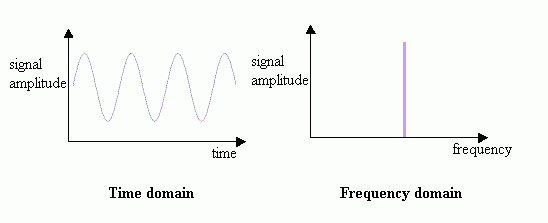The perfect sine wave

In the frequency domain, where things are represented according to frequency on the x-axis, the sine wave is simplicity itself. All its information is concentrated at one frequency. How convenient.

Unfortunately, in nature, things are rarely as simple as a clean sine wave. But wait! There is good news. To a certain approximation, all signals can be broken down into a sum of sine waves of different frequencies. This is called a Fourier transform. Even a gnarly looking square wave can be approximated by a sum of beautiful and easy to handle sine waves. Look at the drawing below.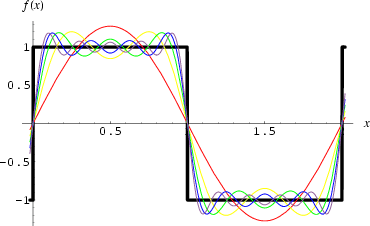A Fourier transform

If one were to add all the nice, smooth sine waves together, the resulting wave would begin to approximate the gnarly square wave. The higher the frequency of the sine waves, the sharper the “corners” of the resulting wave, and the closer it would resemble the original square wave. Note that the amplitude of the sine waves decreases as the frequency goes up. This means that we need less and less additions at the higher frequencies. This is cool, because now we can deal with a series of nice, smooth sine waves instead of the gnarly square wave.

If you look closely, you can see that most of the “information” about the square wave is contained in the first (red) sine wave. It has the same frequency as the square wave, and nearly the same amplitude. It is called the fundamental frequency. The higher frequency sine waves (yellow, green, blue) do contribute some accuracy, but each one adds less and less information to the complete picture. It is the information contained in the energy that is critical in determining how much is enough.

We can apply this idea — that information is contained mainly at certain frequencies — to an ECG. An ECG can be decomposed into a series of nice, smooth sine waves at higher and higher frequencies. The fundamental frequency of an ECG is the patient’s heart rate, usually somewhere around 1 beat per second, or in frequency jargon, 1Hz. The important part is that the preponderance of information for an ECG signal is contained at frequencies below a certain point.

So what is that point? The theory in sampling starts with the frequency range one needs to reproduce. By the standards given by the FDA and their European counterparts IEC/CE, one must go up to 40Hz. The reality is that there is little signal (Read: information) past 25 Hz, and essentially nothing but noise past 60 Hz. As we saw before, one must sample at a minimum of 2X the highest frequency in the signal. (For you audiophiles out there who want to read or know more, this is called the Nyquist rate). That would dictate a system sampling rate of at least 120 Hz. Because of the way things work in the binary electronics world of ones and zeros, the easiest frequency near there is often chosen as 128 Hz (27).

In the DR200/HE, NEMon chose 180 Hz because it is 1) higher than the requirement, and 2) at a multiple of the power line frequency that makes it easier to remove line noise and to be more conservative.

There are a couple of “special” measurements that can be made on ECG data that require a higher resolution. One is HRV (Heart Rate Variability). The clinical threshold for a medical diagnosis starts at .050 seconds, which is 50 ms. Given the sample rate in the DR200/HE of 180 Hz, that gives a resolution of .0055 sec or 5.5 ms. This is a large, safe margin, so HRV measurements using the DR200 recorders are quite reasonable.

Another special measurement is the one for late potentials, sometimes called Signal Averaged ECG (SAECG). As the name implies, these are very tiny signals that are so small that to detect them requires averaging over time in an electrically “quiet” environment. Typically, this has been done in a hospital setting, using an ECG cart. However, with a high enough sample rate and/or enough signal averaging, the same measurements can be accomplished with the DR181 Holter in High Resolution mode, which uses a sample rate of 1440 Hz. The DR181 12L is the only Holter in the world that is certified and qualified to make these measurements.

Subsequent analysis uses the “gold standard” Predictor software algorithm. The signals of interest in this measurement are as high as 250 Hz, so the sample rate of 1440 Hz allows a very safe margin.

Finally, 12-lead Holter recordings are required to have a 100 or 150 Hz bandwidth depending on the standard one is looking at. The higher of the two requires a Nyquist frequency of 300 or so in the DR181 12L capable recorder. NEMon uses 720 Hz (60 Hz * 12) to allow for a significant margin.

The sampling rate is an important number, but above a certain rate, it is pure “specsmanship.” The harmful effects of oversampling (i.e., aliasing) are a topic for another day. In terms of system performance, sampling at higher rates lowers battery life and reduces setup and hold times for components in the recorder. Thus, more is not always better! Sometimes enough is just enough.